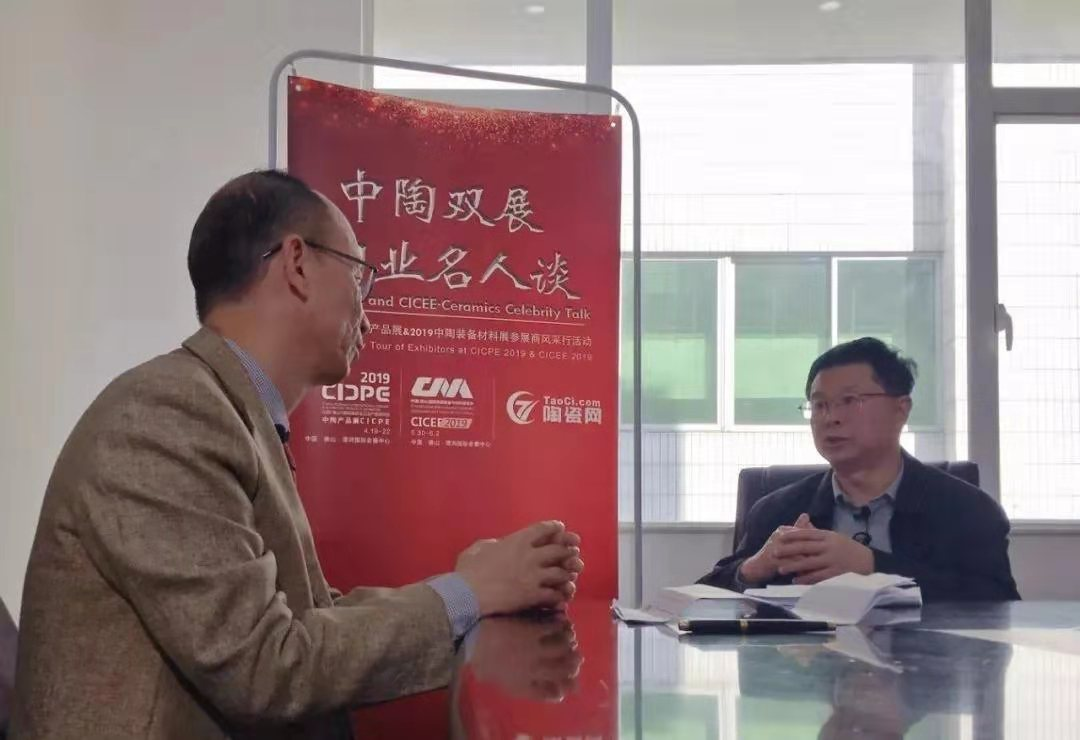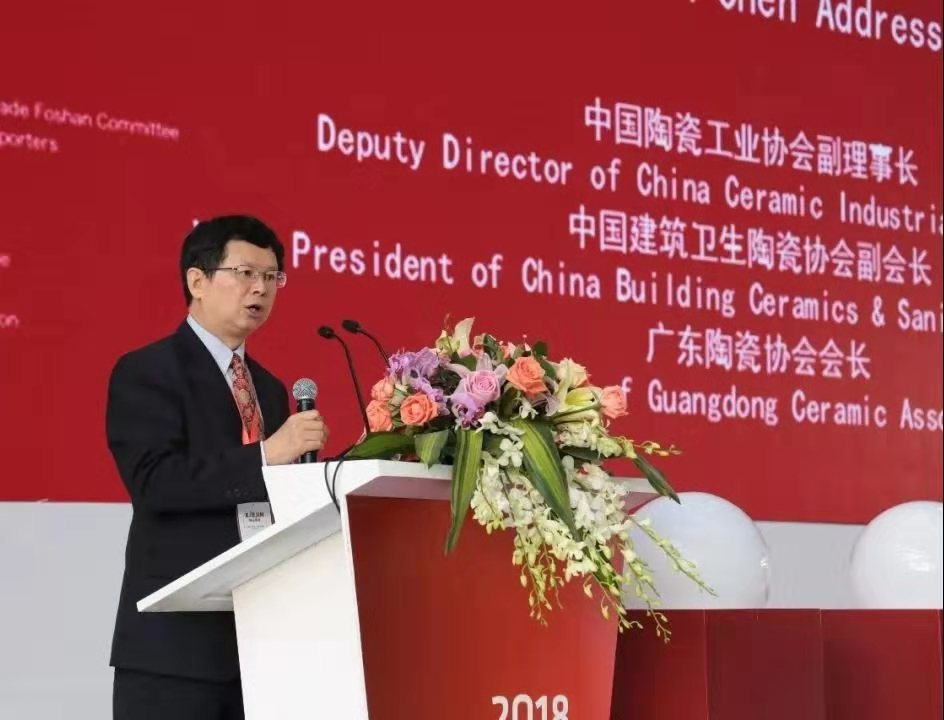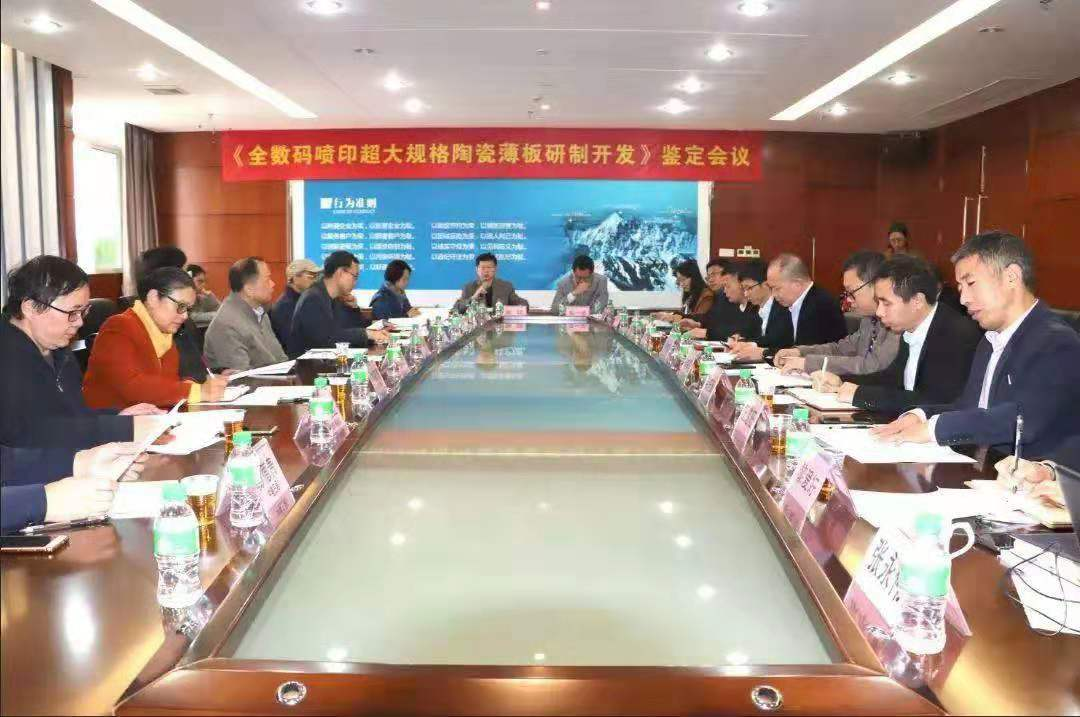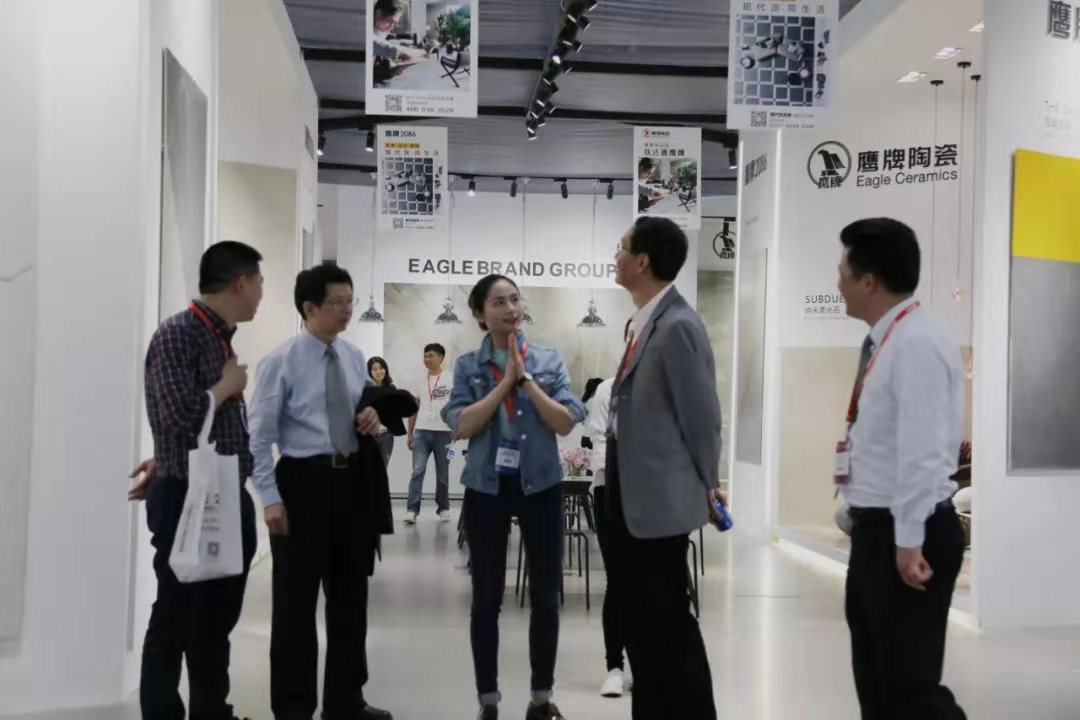|

# 陈环:信心不足企业退出 洗牌是未来发展的需要(上)

2019年1月30日，“中陶双展&陶业名人谈”到访广东陶瓷协会，拜访了陈会长，有幸聆听陈会长站在行业协会的高度，综合陶瓷行业发展轨迹，统筹分析了行业形态，助力企业拨开层层迷雾，选择转型升级的风口，坚定发展的铿锵决心。

采访内容共两期，上述为第一期

第二期精彩继续，敬请留意

以下是采访视频整理内容（内容未经本人核实）：

1

“现时的困难，

是为行业未来健康发展而洗牌”

问：中美贸易摩擦、环保督察严抓等新形势下，部分陶瓷企业出现了倒闭，您认为行业发展是否进入寒冬时期？

进入拐点是肯定的，我不太喜欢用“寒冬”来讲。“寒冬”也不是不可以的，但是我认为可能会影响行业发展的信心。讲一个更明显的例子：很多北方人冬天到海南，甚至到澳洲去过冬，我们这边是寒冬，在另外一个地方可能还是夏天。就是说理解这个寒冬，行业是有希望的，只是说这段时间可能困难多一些，但是可能恰恰就是你的机会，这样来理解我们目前所处的拐点可能对我们的帮助会更大一些。在当前的阶段，无论是城乡的建设或者社会发展，人民群众的生活更美好的追求保证了陶瓷产品始终有比较大的市场需求。

我认为当前的一些困难，是在原来高速发展下，（企业）数量太过庞大（产能严重过剩了），从（行业）自身更健康的发展，淘汰一些，洗洗牌，对于我们未来发展也是一种需要。2

“房地产客流不是说没有，

有些地方甚至需求还很不错”

问：除此以外，房地产集采、电商冲击下，瓷砖的营销渠道和方式在发生急剧而且深刻的变化，参加高端、主流、专业的展会能否为此时的国内陶瓷行业带来什么？

我们认为帮助是很大的。现在我们除了这个行业原来从高速发展到现在相对平稳发展这个拐点，另外也就是你刚才讲的营销的变革，这个痛可能更大一些、更明显一点。原来投资企业，我们说营销因为有强大的经销商队伍，相对来讲简单一些。经销商主要靠零售（可能也做包括房地产、整装等服务），现在发生了改变，很多人都觉得包括建材市场见不到客了，这个可能对信心有很大的打击。

实际上客流不是说没有了（而是零售客少了，精装修、一站式服务的集采、团购多了），房地产虽然有调整但仍在高位，甚至说我们说有一些三四线的城市，甚至是乡镇，还保持比较活跃的程度，那边的需求其实还是不错的。因为实际上的确在大城市的影响大一点，不太好快速赚钱了。如果没有办法适应新的变化，就感觉到比较痛，或者是感觉比较冷，对信心有一些影响。3

“采用优质材料容易定高房价，

这才是房企采购看重的”

这两天广东省人大开会也讲了广东发展形势，接近了10万亿，80%是在珠三角地区。无论是房地产或者是整装，对于品牌质量可能更在乎。现在一些一二线城市的房价已经去到几万块钱（每平方米），增加一两千块应该不是太大的变化。但是一两千块钱对于材料，特别对于陶瓷来讲可能已经是很大了，如果用了品牌的材料容易把房价定得高一点，这个影响他们是比较在乎的。

一些著名的房地产企业曾经也吃过亏，比如说万科当初的毒地板事件，对他们的影响是非常大，不单只是价格可能下降一两千块钱的问题，整个万科的品牌都会受到冲击。所以他们对于质量要做到万无一失。

但是也讲创新，新产品也会对他们有非常强的吸引力。原来可能同质化还有一定的市场，无非通过性价比，比别人高一点就有市场，当要综合考虑价格问题的时候，把所有都算到里面去的话，他们宁可贵一点点也要选择有品牌的，或者是新产品，所以说这些我都觉得对我们行业来讲是一个很大的改变，我们要适应新的发展的形势。4

“原来斗性价比，现在到展会斗创新”

中陶产品展就是顺应社会发展需要搭建的（平台），另外一个方面觉得未来我们要更多的国际交流。现在很多产品的设计是以国外理念为主，实际上我们中国人有5000年文化，这种创新设计的产品应该说还是比较少的，结合更多本土文化和深厚的历史底蕴，融入更多的中国元素，这个也是我们未来开发产品的潜力。

比如我们5000年历史的陶瓷，是釉的变化（是重要方面）。如很多民窑的釉本身在外国人心目是一个很神圣的东西，这些都是我们可以在陶瓷砖上应用。这些其实我们还是做得不够的，所以通过这个展也是引导行业来创新发展。

原来还可以斗性价比，你做这个我也做这个，在展会经济（时代）这种机会就越来越少了，不可能拿仿冒的东西去那里摆，大家竞争就是要讲创新，讲设计理念，这些都是引导行业在未来新时期更加强调创新发展。

中陶产品展不纯粹是一个展，而是一个大的平台，一个国际化的平台。意大利、西班牙、（甚至）东南亚国家都有了，还要继续扩大这种国际化平台的交流。同台竞技，像奥林匹克一样，可以解决外国品牌说我们知识产权保护问题，引导企业往这方面走。

企业打假成本太高。国家要管是要通过法律（而诉讼是）比较漫长，所以展会更有利于知识产权的保护。因为产品在展示的时候（假冒的不能摆）可能就影响了房地产企业的采购，或者是整装的采购。甚至说以后展会就是房地产更多了解材料企业，决定一年主要采购方向的时机。展会来做引导创新，培育材料企业一起做品牌。也通过跟国外深入的同台竞技，真正激发中国企业创新。

另外还有一个很重要的，更能让外国人看到我们的产品，看见我们的实力，以后来做生意。你有特色的东西，外国人会更容易补充到他们体系里。这种交流合作创造了非常好的环境。5

“退出是因信心不足，集中更能稳定发展”

一部分信心不足的企业退出我也觉得不是什么坏事，他肯定就觉得还是很痛苦的，脱离痛苦也是一种快乐。对陶瓷行业来讲，通过这种方式提高了集中度，让有信心的企业能够做的更稳定一些，也是好事。所以说在这种环境底下，我们就是刚才讲的要引导有信心的企业在这方面的发展，更有利于他在未来获得更大的回报。

（未完待续）

下期精彩继续

陈会长又将带来哪些干货

诚邀您继续关注

“中陶双展&陶业名人谈”

`声明：本文由入驻焦点开放平台的作者撰写，除焦点官方账号外，观点仅代表作者本人，不代表焦点立场错误信息举报电话： 400-099-0099，邮箱：jubao@vip.sohu.com，或点此进行意见反馈，或点此进行举报投诉。`A B C D E F G H J K L M N P Q R S T W X Y Z
A - B - C - D - E
• A
• 鞍山
• 安庆
• 安阳
• 安顺
• 安康
• 澳门
• B
• 北京
• 保定
• 包头
• 巴彦淖尔
• 本溪
• 蚌埠
• 亳州
• 滨州
• 北海
• 百色
• 巴中
• 毕节
• 保山
• 宝鸡
• 白银
• 巴州
• C
• 承德
• 沧州
• 长治
• 赤峰
• 朝阳
• 长春
• 常州
• 滁州
• 池州
• 长沙
• 常德
• 郴州
• 潮州
• 崇左
• 重庆
• 成都
• 楚雄
• 昌都
• 慈溪
• 常熟
• D
• 大同
• 大连
• 丹东
• 大庆
• 东营
• 德州
• 东莞
• 德阳
• 达州
• 大理
• 德宏
• 定西
• 儋州
• 东平
• E
• 鄂尔多斯
• 鄂州
• 恩施
F - G - H - I - J
• F
• 抚顺
• 阜新
• 阜阳
• 福州
• 抚州
• 佛山
• 防城港
• G
• 赣州
• 广州
• 桂林
• 贵港
• 广元
• 广安
• 贵阳
• 固原
• H
• 邯郸
• 衡水
• 呼和浩特
• 呼伦贝尔
• 葫芦岛
• 哈尔滨
• 黑河
• 淮安
• 杭州
• 湖州
• 合肥
• 淮南
• 淮北
• 黄山
• 菏泽
• 鹤壁
• 黄石
• 黄冈
• 衡阳
• 怀化
• 惠州
• 河源
• 贺州
• 河池
• 海口
• 红河
• 汉中
• 海东
• 怀来
• I
• J
• 晋中
• 锦州
• 吉林
• 鸡西
• 佳木斯
• 嘉兴
• 金华
• 景德镇
• 九江
• 吉安
• 济南
• 济宁
• 焦作
• 荆门
• 荆州
• 江门
• 揭阳
• 金昌
• 酒泉
• 嘉峪关
K - L - M - N - P
• K
• 开封
• 昆明
• 昆山
• L
• 廊坊
• 临汾
• 辽阳
• 连云港
• 丽水
• 六安
• 龙岩
• 莱芜
• 临沂
• 聊城
• 洛阳
• 漯河
• 娄底
• 柳州
• 来宾
• 泸州
• 乐山
• 六盘水
• 丽江
• 临沧
• 拉萨
• 林芝
• 兰州
• 陇南
• M
• 牡丹江
• 马鞍山
• 茂名
• 梅州
• 绵阳
• 眉山
• N
• 南京
• 南通
• 宁波
• 南平
• 宁德
• 南昌
• 南阳
• 南宁
• 内江
• 南充
• P
• 盘锦
• 莆田
• 平顶山
• 濮阳
• 攀枝花
• 普洱
• 平凉
Q - R - S - T - W
• Q
• 秦皇岛
• 齐齐哈尔
• 衢州
• 泉州
• 青岛
• 清远
• 钦州
• 黔南
• 曲靖
• 庆阳
• R
• 日照
• 日喀则
• S
• 石家庄
• 沈阳
• 双鸭山
• 绥化
• 上海
• 苏州
• 宿迁
• 绍兴
• 宿州
• 三明
• 上饶
• 三门峡
• 商丘
• 十堰
• 随州
• 邵阳
• 韶关
• 深圳
• 汕头
• 汕尾
• 三亚
• 三沙
• 遂宁
• 山南
• 商洛
• 石嘴山
• T
• 天津
• 唐山
• 太原
• 通辽
• 铁岭
• 泰州
• 台州
• 铜陵
• 泰安
• 铜仁
• 铜川
• 天水
• 天门
• W
• 乌海
• 乌兰察布
• 无锡
• 温州
• 芜湖
• 潍坊
• 威海
• 武汉
• 梧州
• 渭南
• 武威
• 吴忠
• 乌鲁木齐
X - Y - Z
• X
• 邢台
• 徐州
• 宣城
• 厦门
• 新乡
• 许昌
• 信阳
• 襄阳
• 孝感
• 咸宁
• 湘潭
• 湘西
• 西双版纳
• 西安
• 咸阳
• 西宁
• 仙桃
• 西昌
• Y
• 运城
• 营口
• 盐城
• 扬州
• 鹰潭
• 宜春
• 烟台
• 宜昌
• 岳阳
• 益阳
• 永州
• 阳江
• 云浮
• 玉林
• 宜宾
• 雅安
• 玉溪
• 延安
• 榆林
• 银川
• Z
• 张家口
• 镇江
• 舟山
• 漳州
• 淄博
• 枣庄
• 郑州
• 周口
• 驻马店
• 株洲
• 张家界
• 珠海
• 湛江
• 肇庆
• 中山
• 自贡
• 资阳
• 遵义
• 昭通
• 张掖
• 中卫

1室1厅1厨1卫1阳台

1
2
3
4
5

0
1
2

1

1

0
1
2
3报名成功，资料已提交审核A B C D E F G H J K L M N P Q R S T W X Y Z
A - B - C - D - E
• A
• 鞍山
• 安庆
• 安阳
• 安顺
• 安康
• 澳门
• B
• 北京
• 保定
• 包头
• 巴彦淖尔
• 本溪
• 蚌埠
• 亳州
• 滨州
• 北海
• 百色
• 巴中
• 毕节
• 保山
• 宝鸡
• 白银
• 巴州
• C
• 承德
• 沧州
• 长治
• 赤峰
• 朝阳
• 长春
• 常州
• 滁州
• 池州
• 长沙
• 常德
• 郴州
• 潮州
• 崇左
• 重庆
• 成都
• 楚雄
• 昌都
• 慈溪
• 常熟
• D
• 大同
• 大连
• 丹东
• 大庆
• 东营
• 德州
• 东莞
• 德阳
• 达州
• 大理
• 德宏
• 定西
• 儋州
• 东平
• E
• 鄂尔多斯
• 鄂州
• 恩施
F - G - H - I - J
• F
• 抚顺
• 阜新
• 阜阳
• 福州
• 抚州
• 佛山
• 防城港
• G
• 赣州
• 广州
• 桂林
• 贵港
• 广元
• 广安
• 贵阳
• 固原
• H
• 邯郸
• 衡水
• 呼和浩特
• 呼伦贝尔
• 葫芦岛
• 哈尔滨
• 黑河
• 淮安
• 杭州
• 湖州
• 合肥
• 淮南
• 淮北
• 黄山
• 菏泽
• 鹤壁
• 黄石
• 黄冈
• 衡阳
• 怀化
• 惠州
• 河源
• 贺州
• 河池
• 海口
• 红河
• 汉中
• 海东
• 怀来
• I
• J
• 晋中
• 锦州
• 吉林
• 鸡西
• 佳木斯
• 嘉兴
• 金华
• 景德镇
• 九江
• 吉安
• 济南
• 济宁
• 焦作
• 荆门
• 荆州
• 江门
• 揭阳
• 金昌
• 酒泉
• 嘉峪关
K - L - M - N - P
• K
• 开封
• 昆明
• 昆山
• L
• 廊坊
• 临汾
• 辽阳
• 连云港
• 丽水
• 六安
• 龙岩
• 莱芜
• 临沂
• 聊城
• 洛阳
• 漯河
• 娄底
• 柳州
• 来宾
• 泸州
• 乐山
• 六盘水
• 丽江
• 临沧
• 拉萨
• 林芝
• 兰州
• 陇南
• M
• 牡丹江
• 马鞍山
• 茂名
• 梅州
• 绵阳
• 眉山
• N
• 南京
• 南通
• 宁波
• 南平
• 宁德
• 南昌
• 南阳
• 南宁
• 内江
• 南充
• P
• 盘锦
• 莆田
• 平顶山
• 濮阳
• 攀枝花
• 普洱
• 平凉
Q - R - S - T - W
• Q
• 秦皇岛
• 齐齐哈尔
• 衢州
• 泉州
• 青岛
• 清远
• 钦州
• 黔南
• 曲靖
• 庆阳
• R
• 日照
• 日喀则
• S
• 石家庄
• 沈阳
• 双鸭山
• 绥化
• 上海
• 苏州
• 宿迁
• 绍兴
• 宿州
• 三明
• 上饶
• 三门峡
• 商丘
• 十堰
• 随州
• 邵阳
• 韶关
• 深圳
• 汕头
• 汕尾
• 三亚
• 三沙
• 遂宁
• 山南
• 商洛
• 石嘴山
• T
• 天津
• 唐山
• 太原
• 通辽
• 铁岭
• 泰州
• 台州
• 铜陵
• 泰安
• 铜仁
• 铜川
• 天水
• 天门
• W
• 乌海
• 乌兰察布
• 无锡
• 温州
• 芜湖
• 潍坊
• 威海
• 武汉
• 梧州
• 渭南
• 武威
• 吴忠
• 乌鲁木齐
X - Y - Z
• X
• 邢台
• 徐州
• 宣城
• 厦门
• 新乡
• 许昌
• 信阳
• 襄阳
• 孝感
• 咸宁
• 湘潭
• 湘西
• 西双版纳
• 西安
• 咸阳
• 西宁
• 仙桃
• 西昌
• Y
• 运城
• 营口
• 盐城
• 扬州
• 鹰潭
• 宜春
• 烟台
• 宜昌
• 岳阳
• 益阳
• 永州
• 阳江
• 云浮
• 玉林
• 宜宾
• 雅安
• 玉溪
• 延安
• 榆林
• 银川
• Z
• 张家口
• 镇江
• 舟山
• 漳州
• 淄博
• 枣庄
• 郑州
• 周口
• 驻马店
• 株洲
• 张家界
• 珠海
• 湛江
• 肇庆
• 中山
• 自贡
• 资阳
• 遵义
• 昭通
• 张掖
• 中卫• 手机• 分享
• 设计
免费设计
• 计算器
装修计算器
• 入驻
合作入驻
• 联系
联系我们
• 置顶
返回顶部